# RS Aggarwal Solutions for Class 9 Chapter 12: Circles Exercise 12C

## RS Aggarwal Solutions for Class 9 Maths Exercise 12C PDF

Geometrical structures like Circles are of greater importance in order to understand some of the fundamental concepts in Mathematics. The solutions are done with the aim of making the students comfortable in solving exercise wise problems. This is the third exercise of Chapter 12 which contains solutions designed by subject experts at BYJU’S. The solutions are explained in simple language which match the understanding capacity of students. This exercise has problems which are solved based on cyclic quadrilaterals. RS Aggarwal Solutions for Class 9 Maths Chapter 12 Circles Exercise 12C are provided here.

## RS Aggarwal Solutions for Class 9 Chapter 12: Circles Exercise 12C Download PDF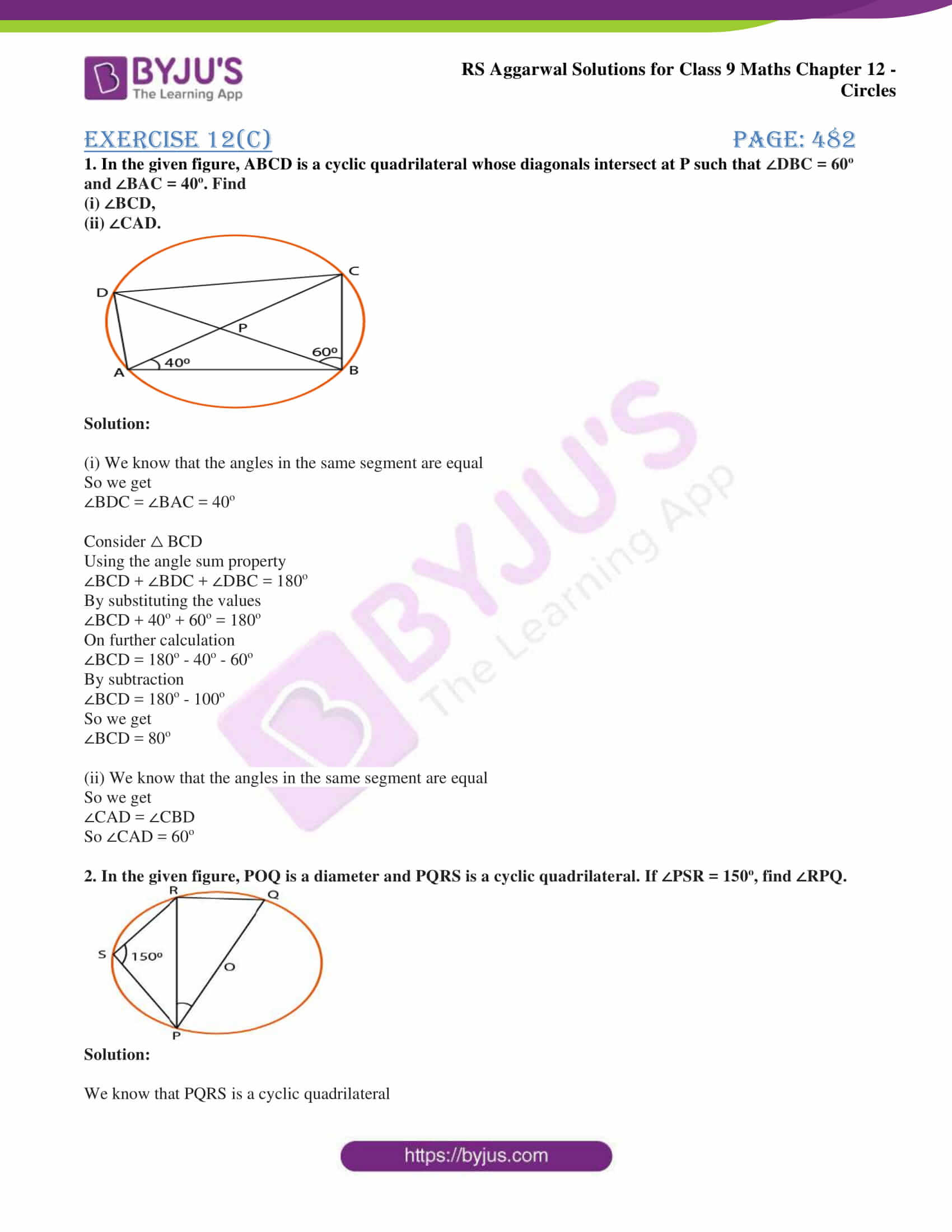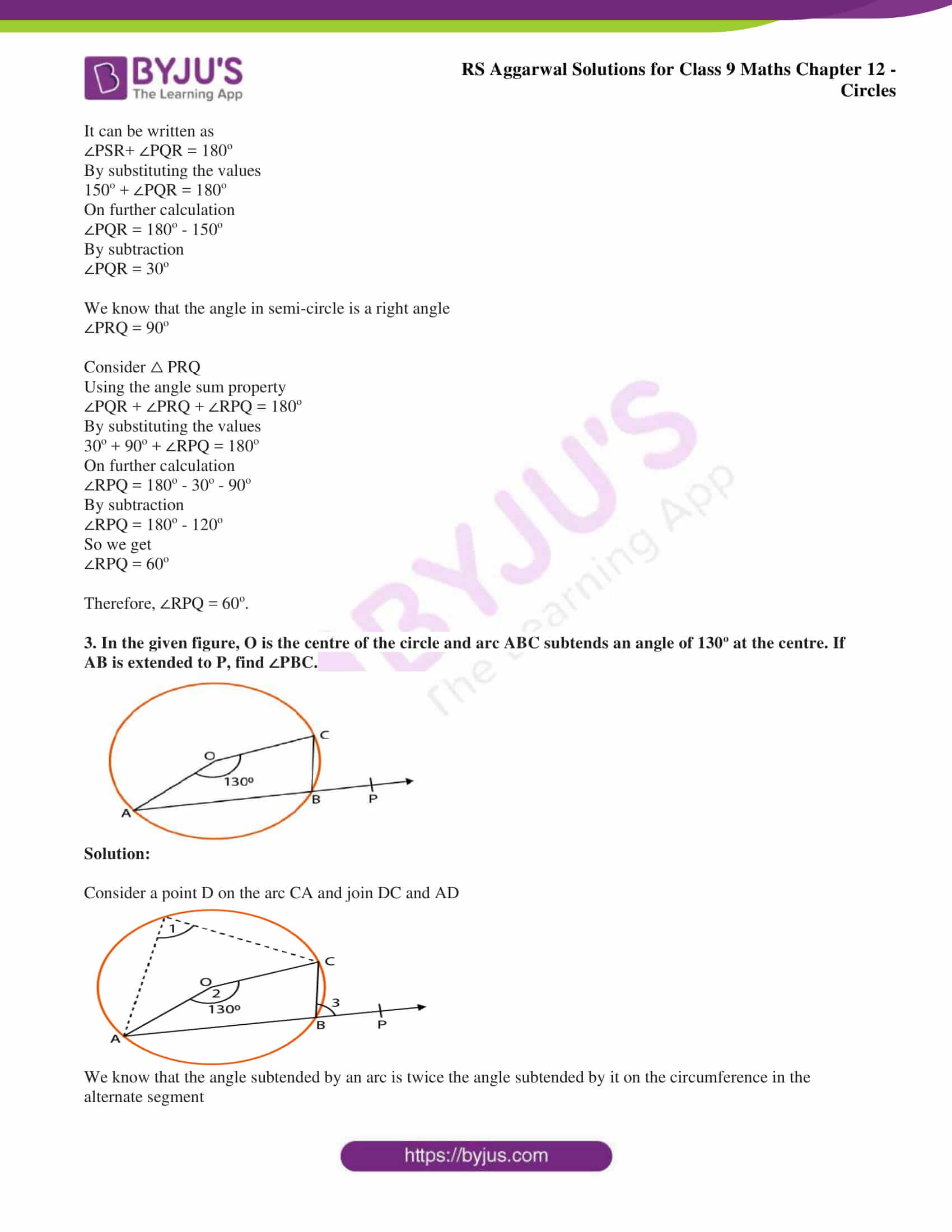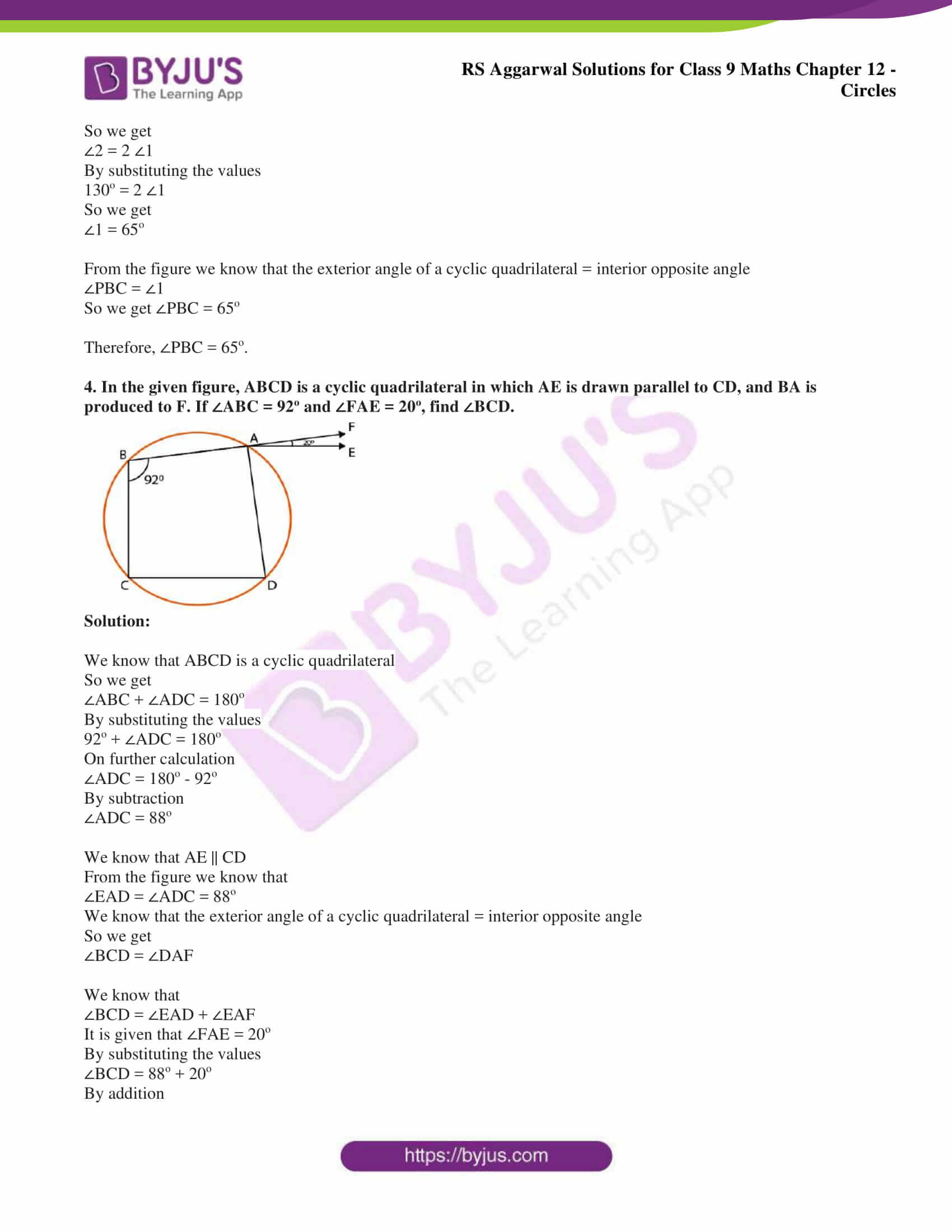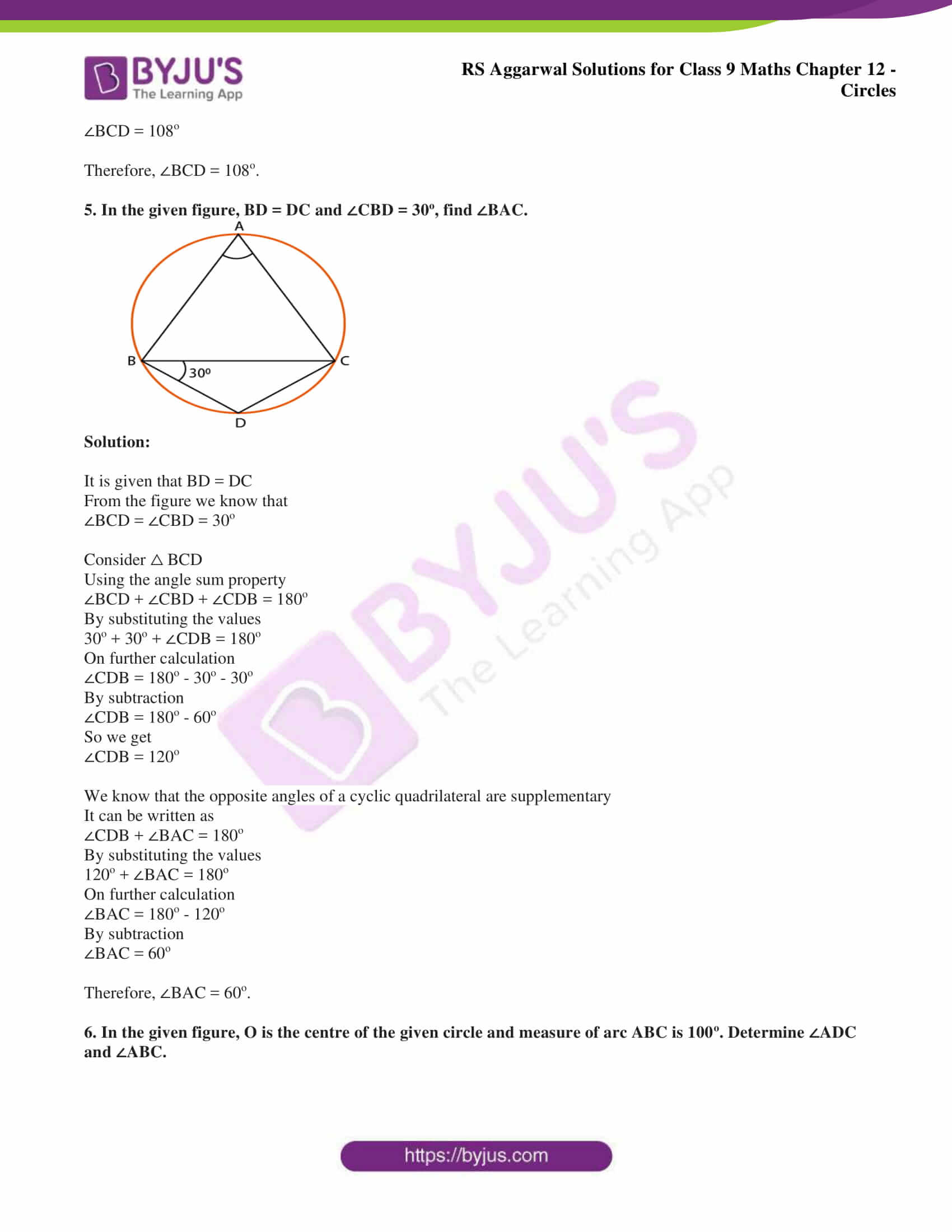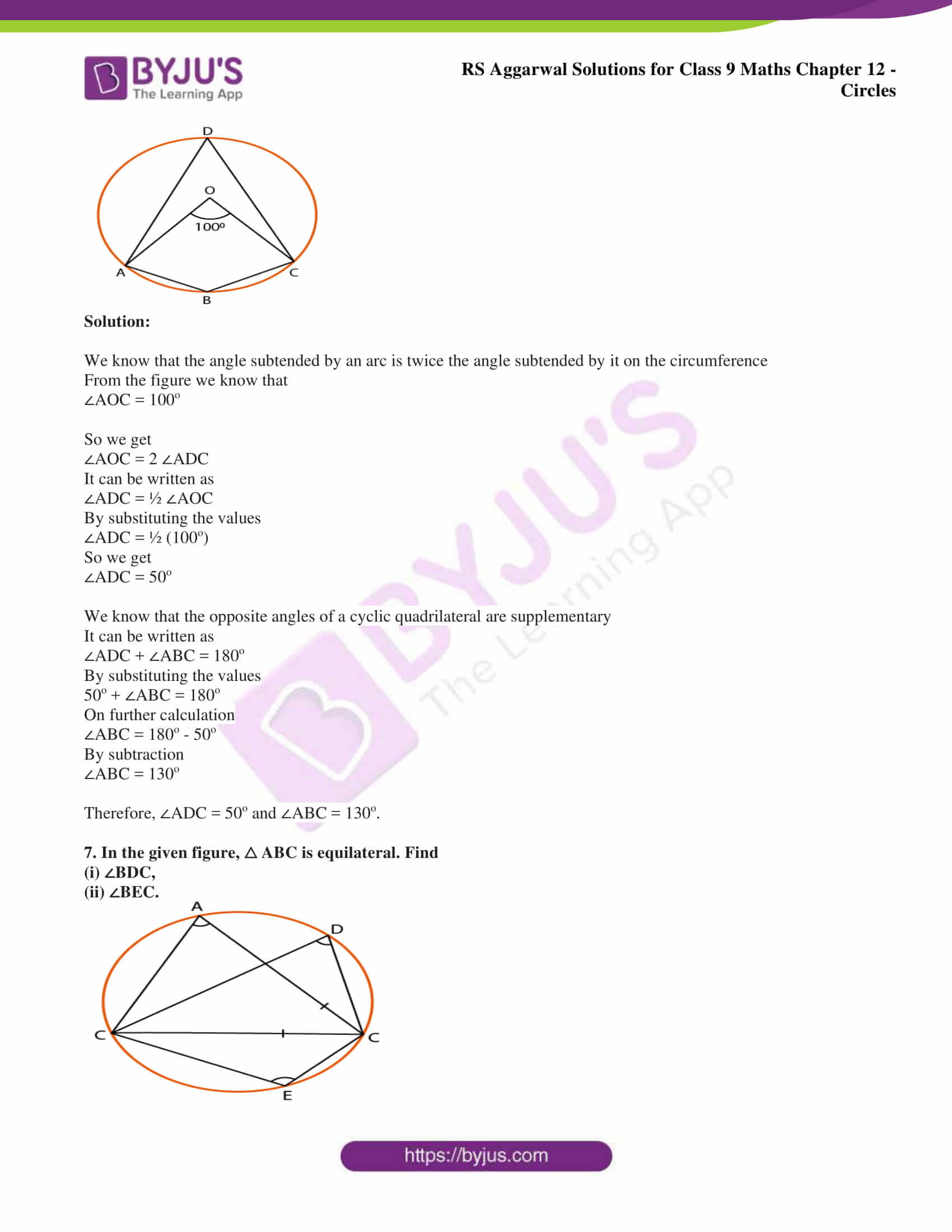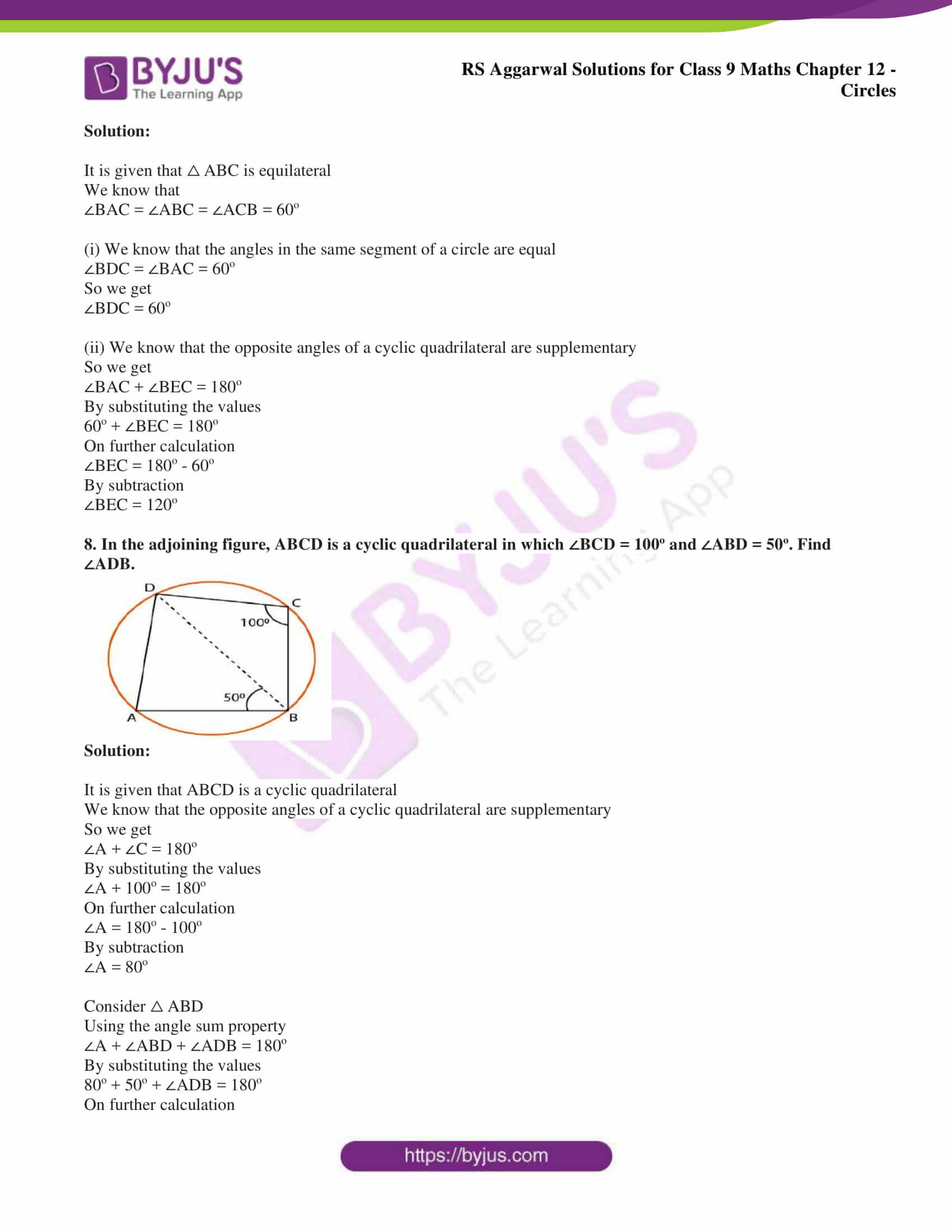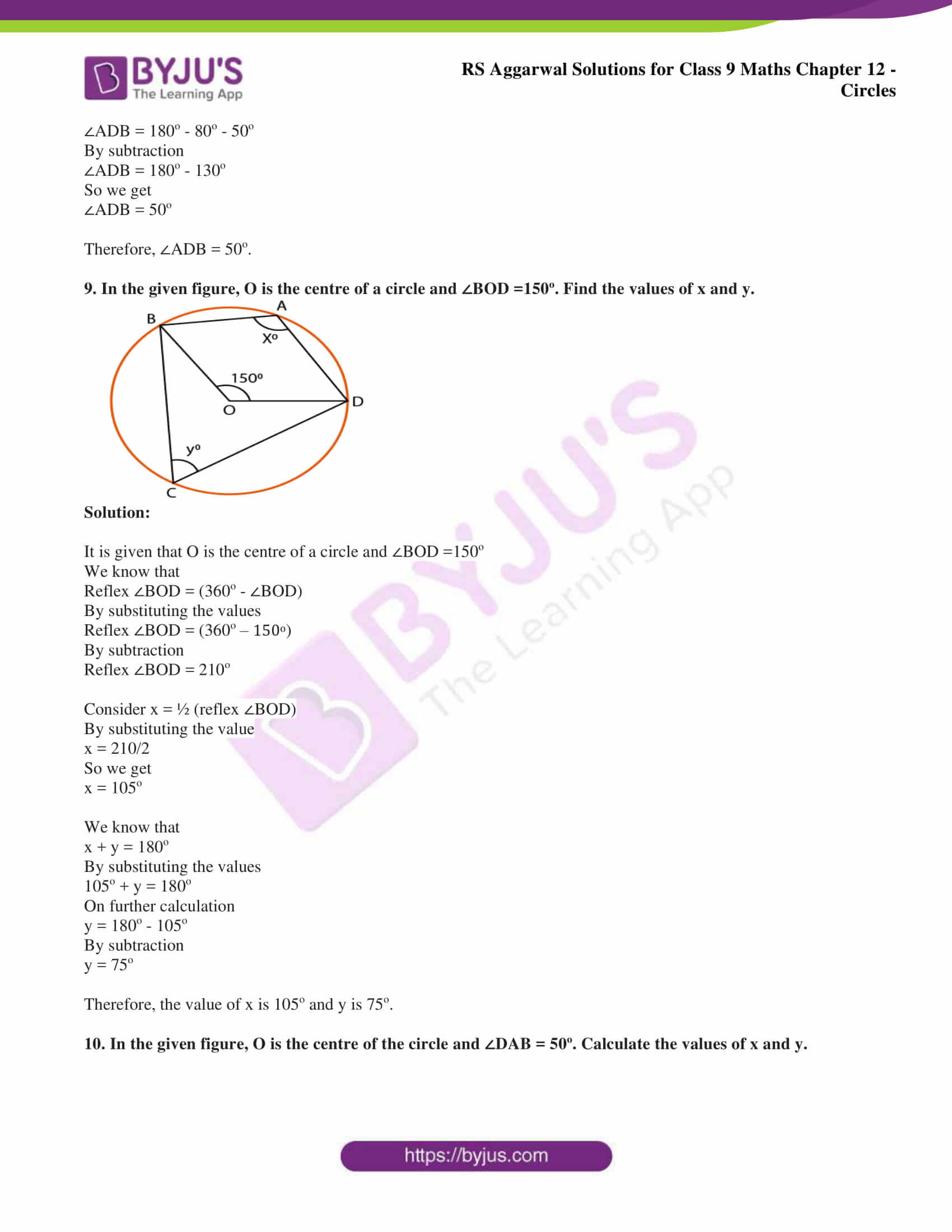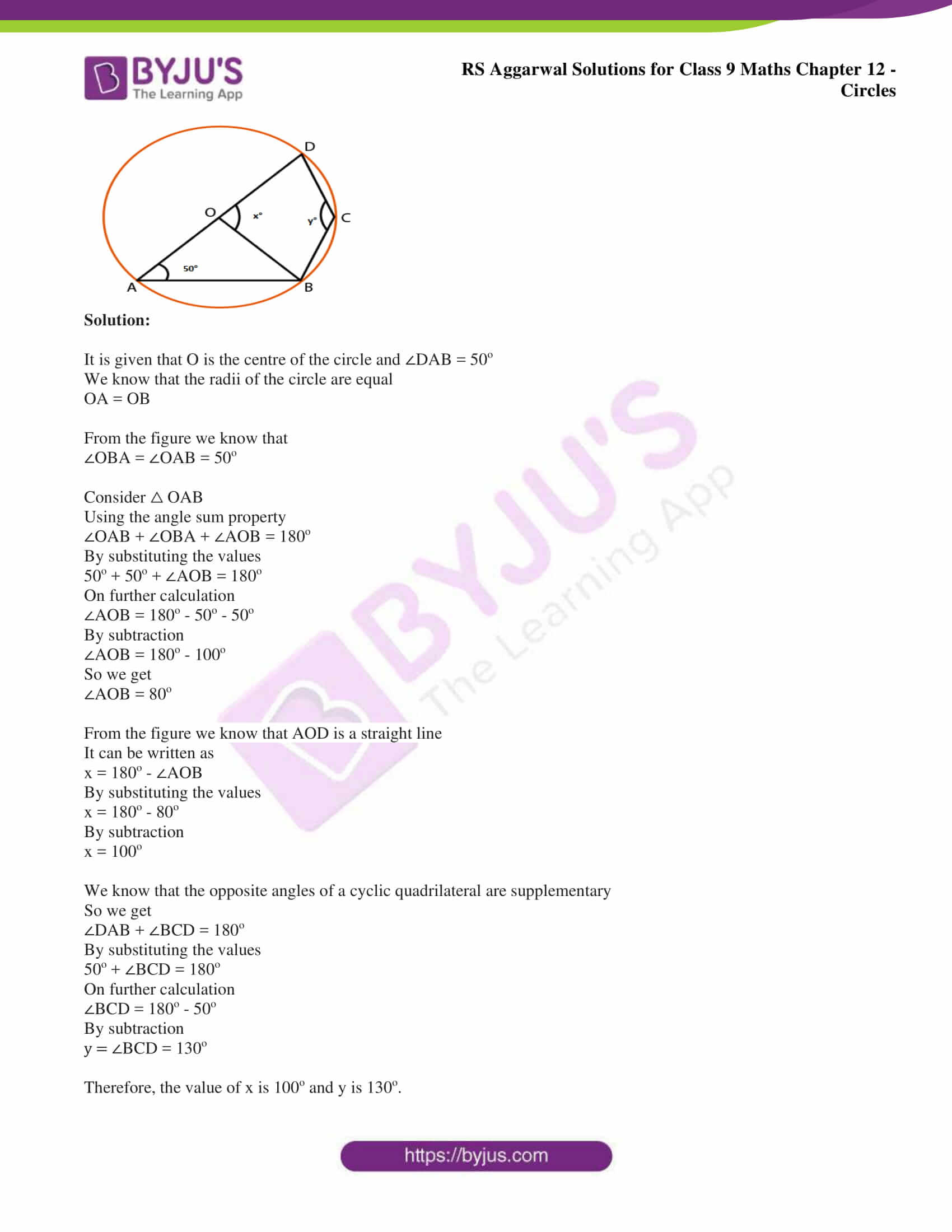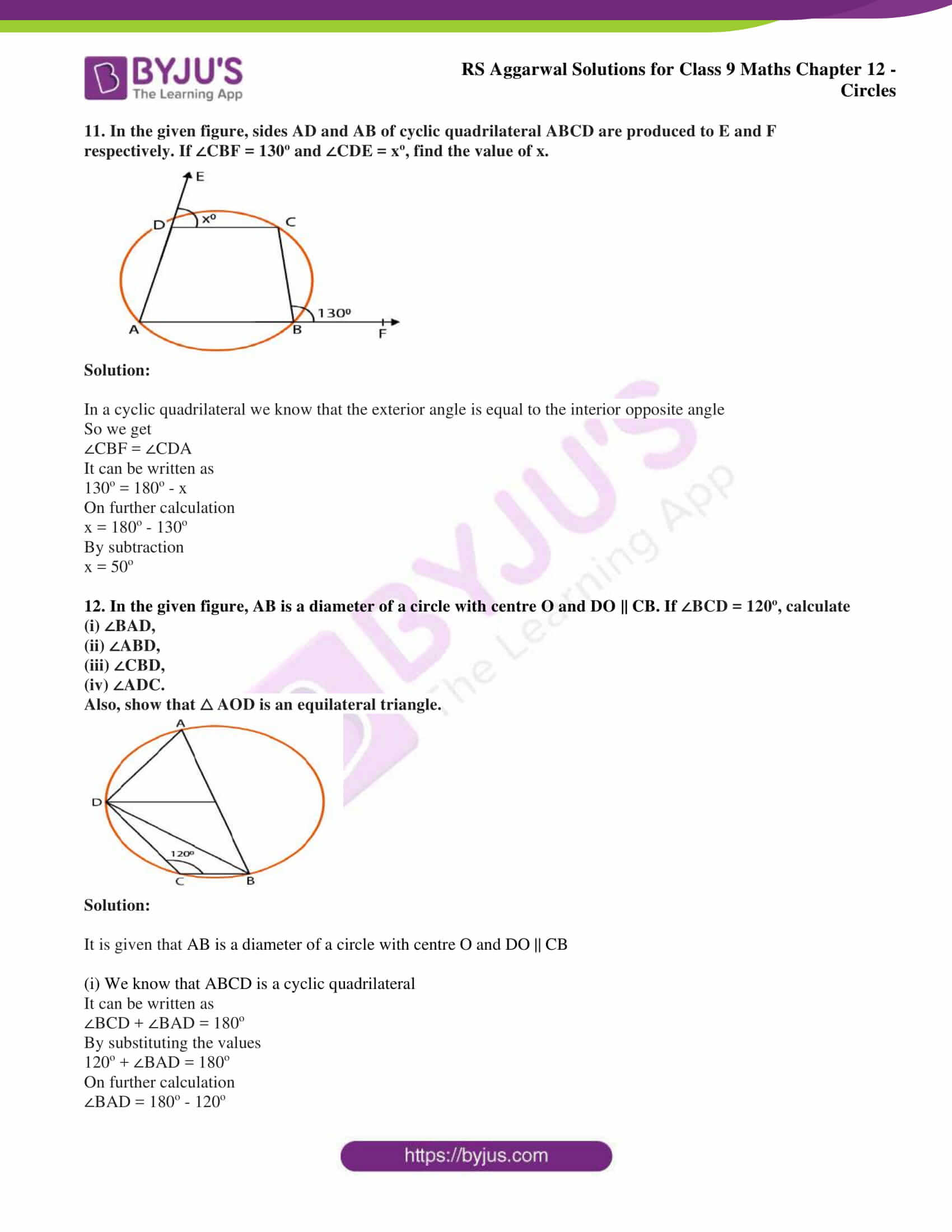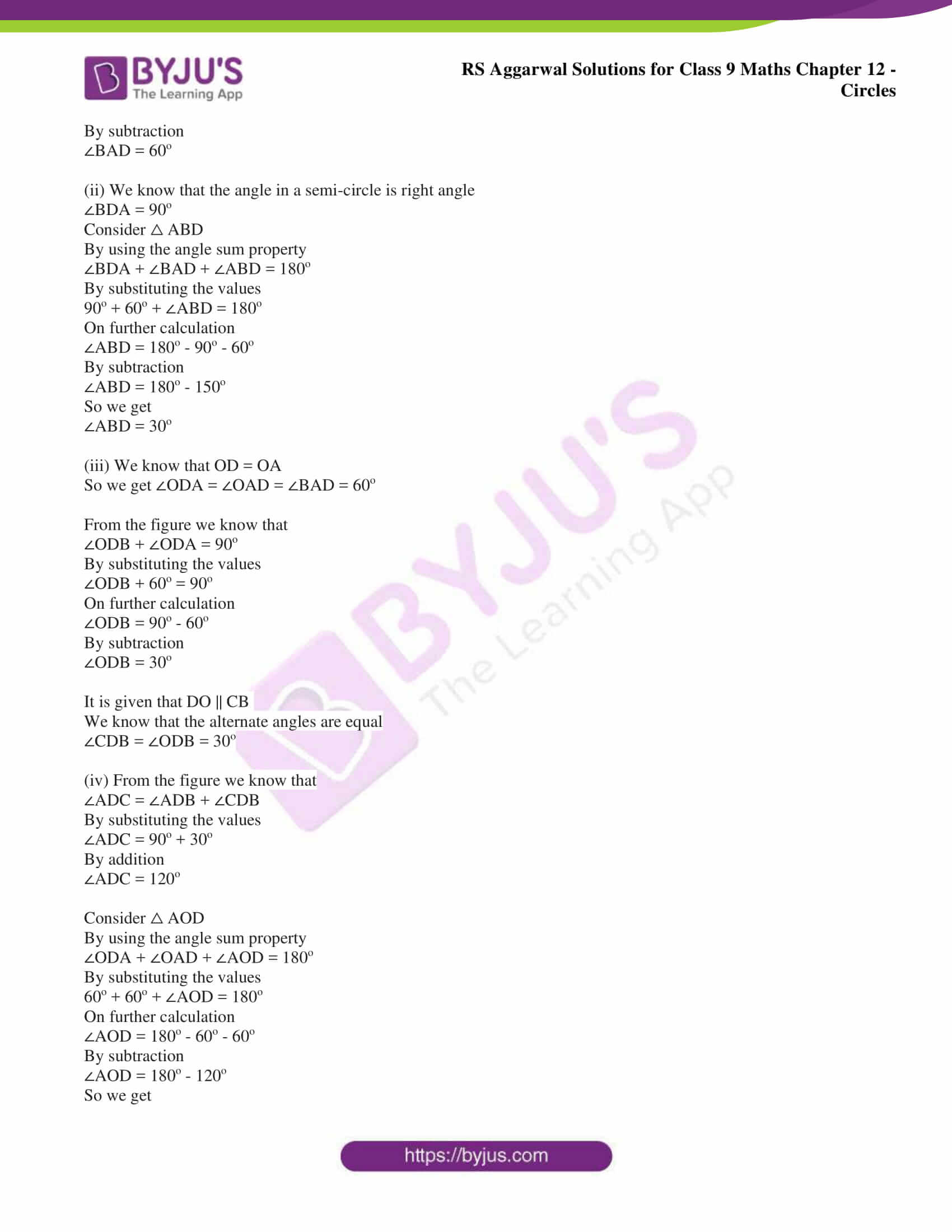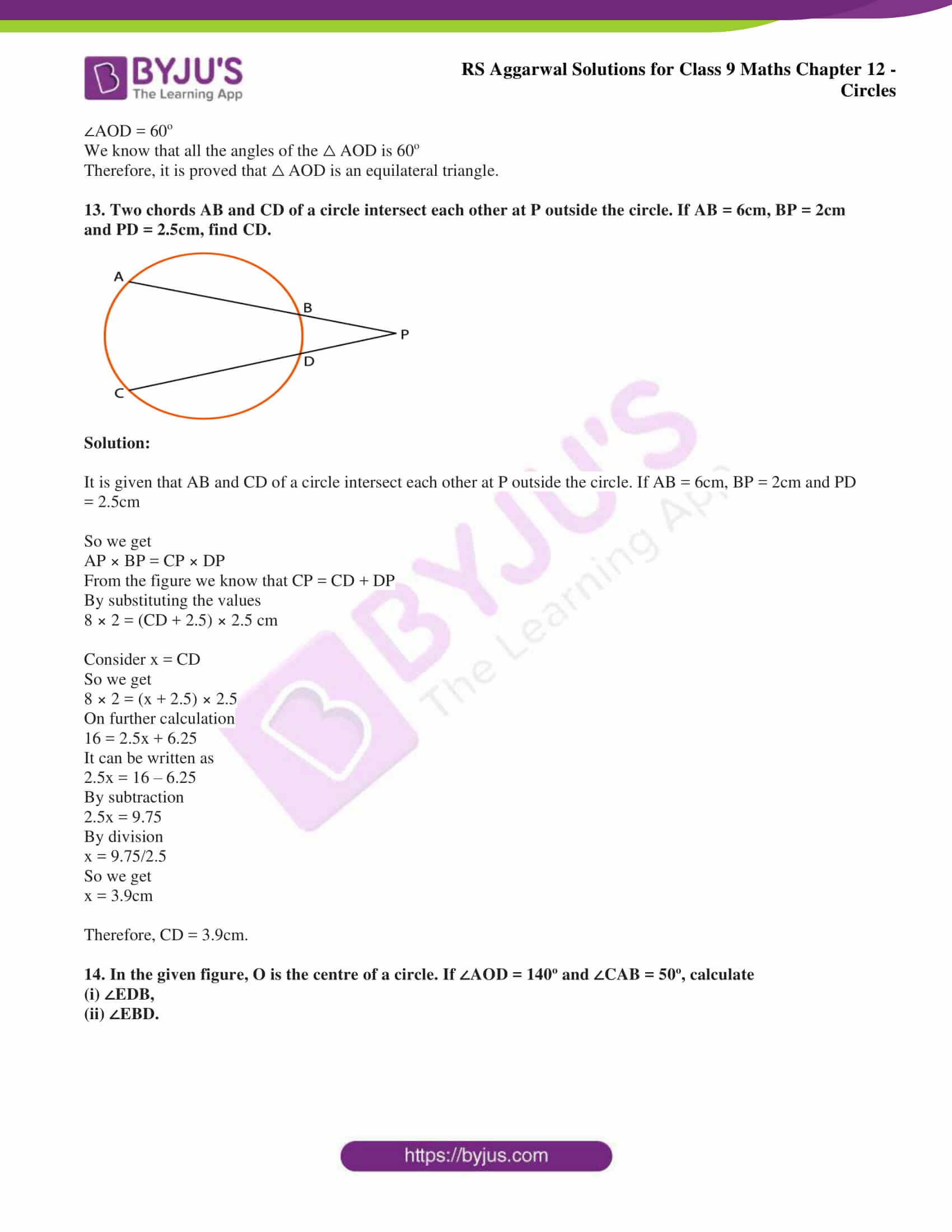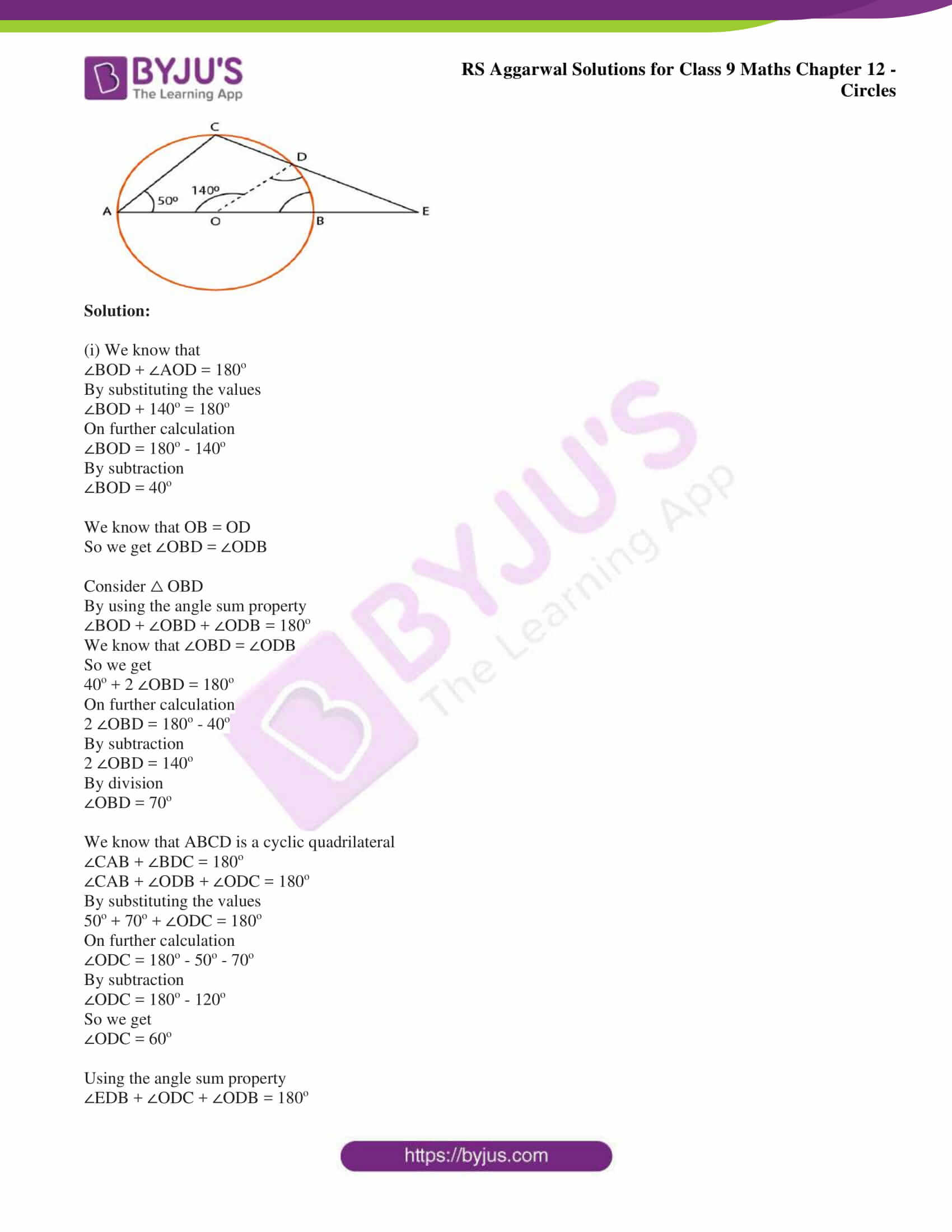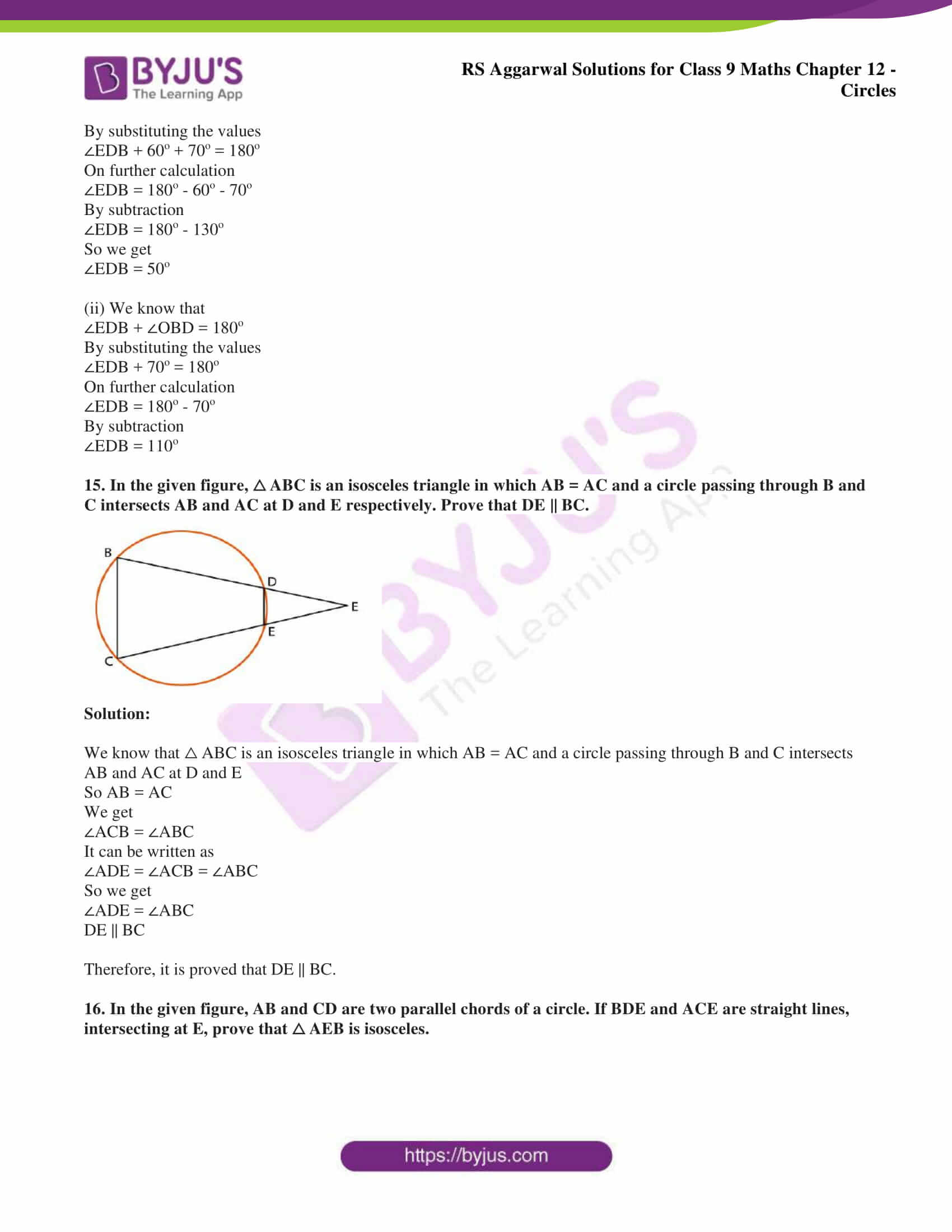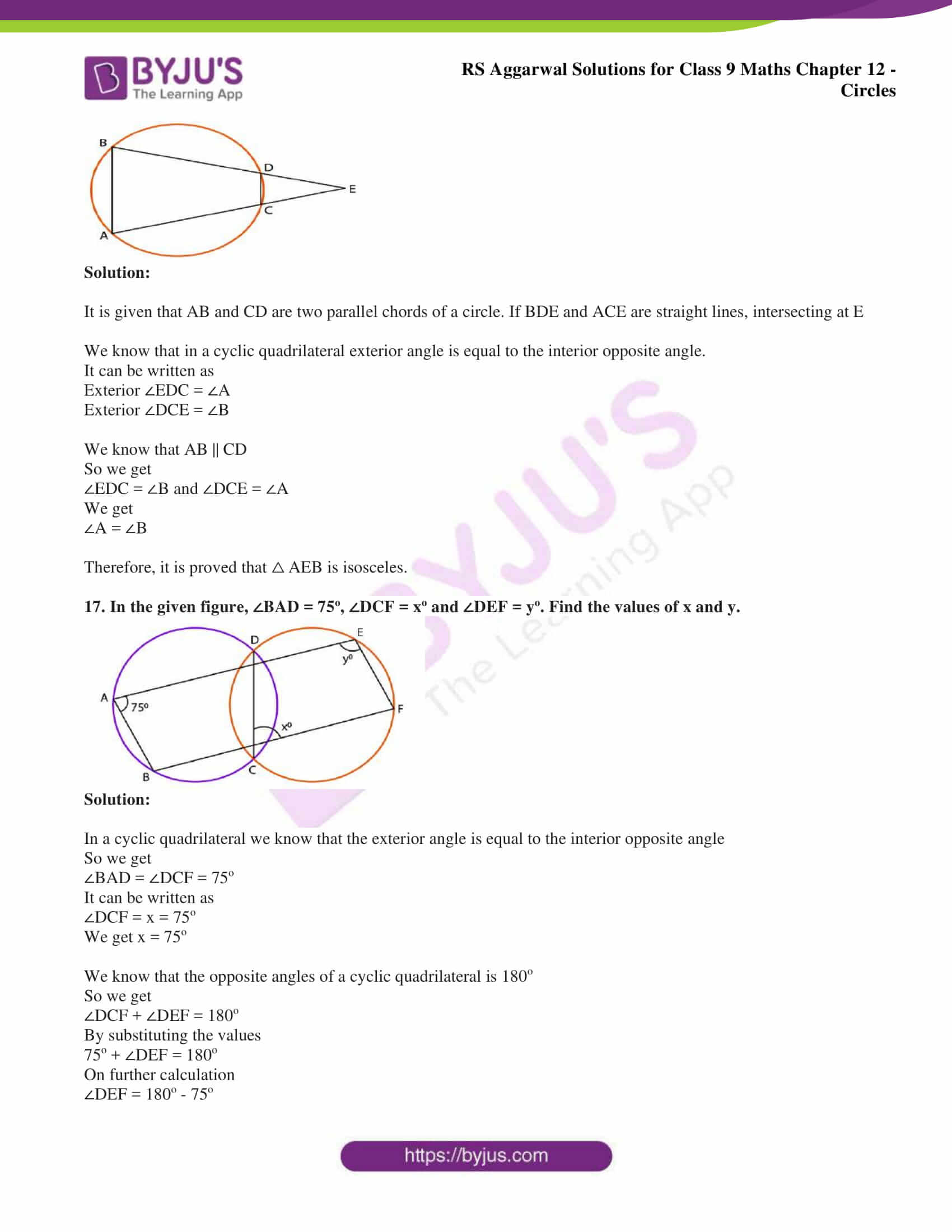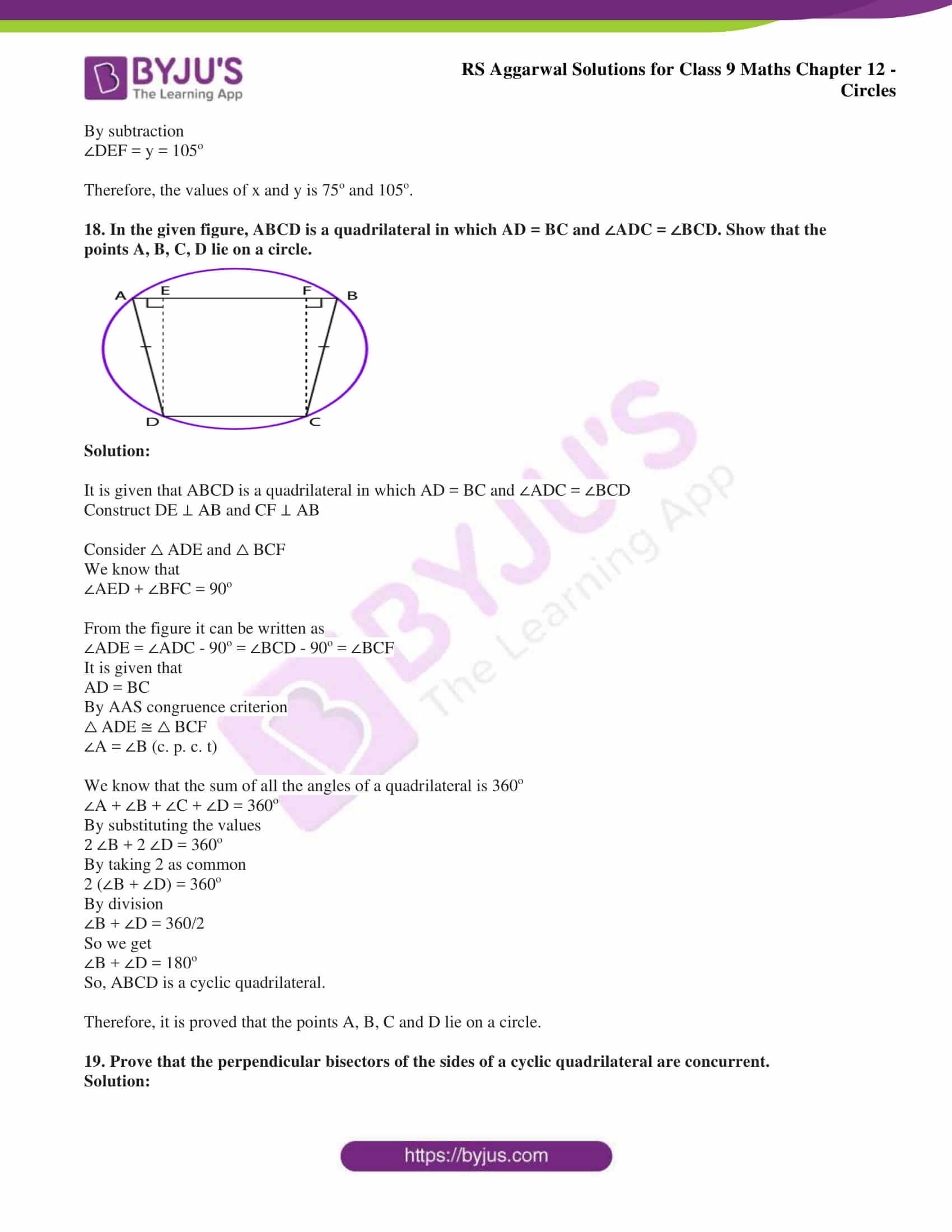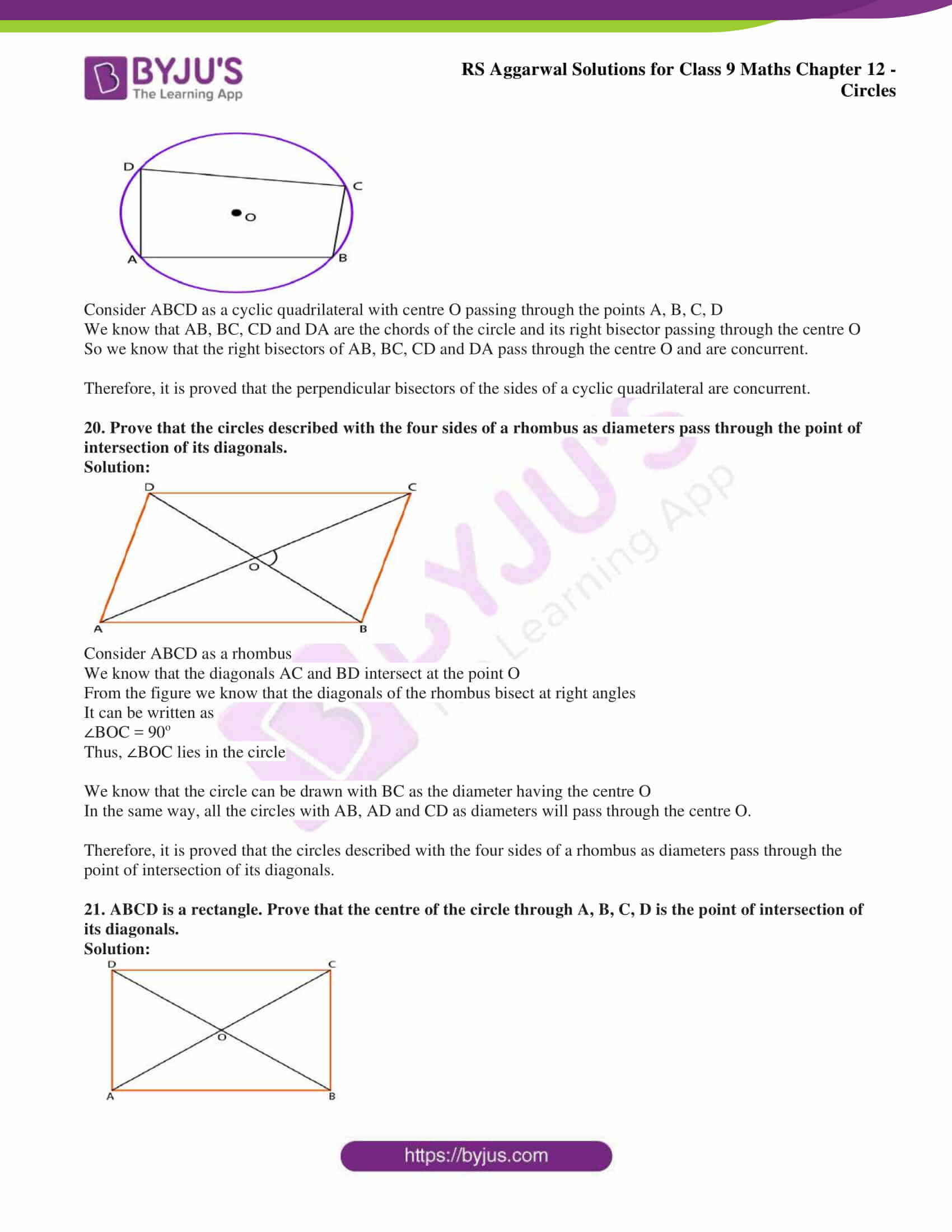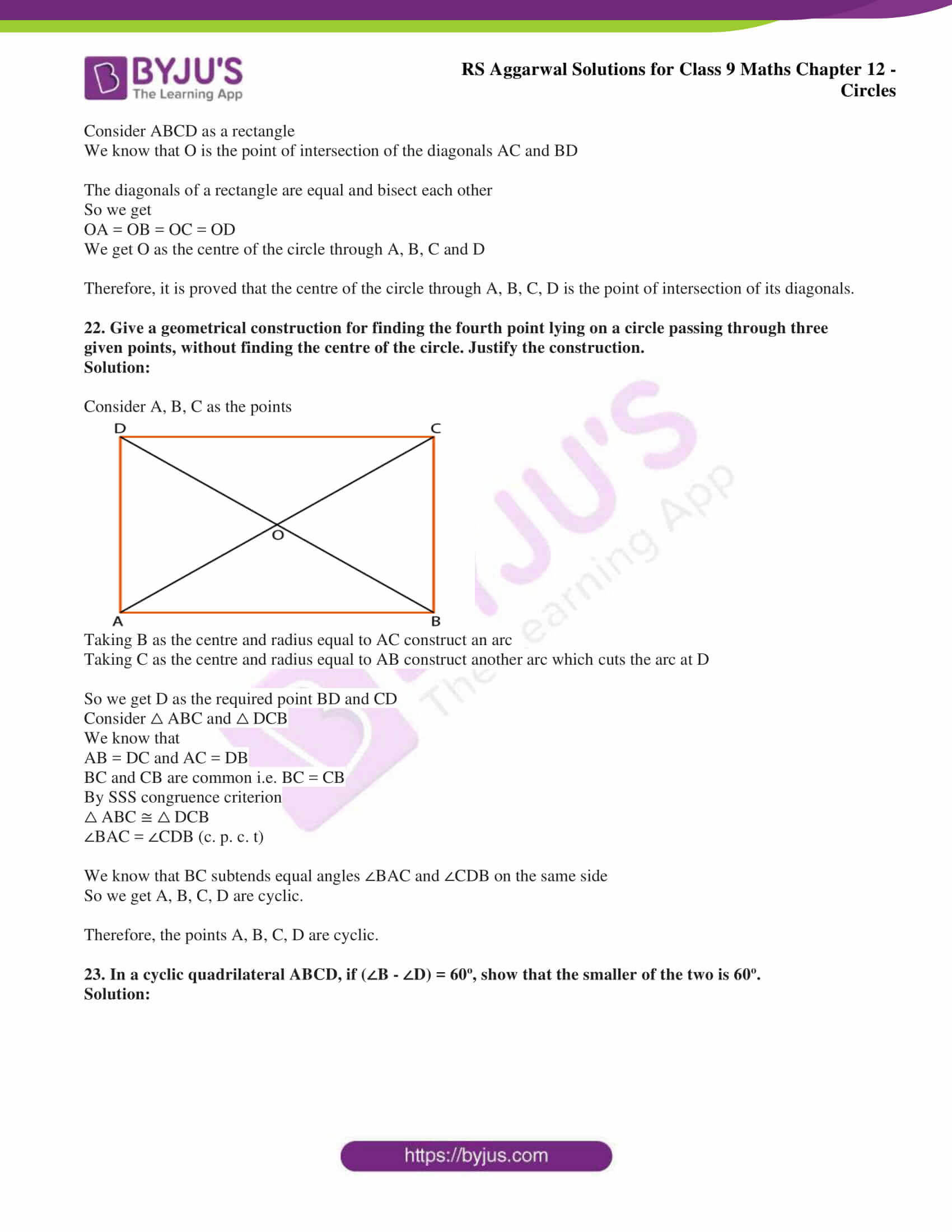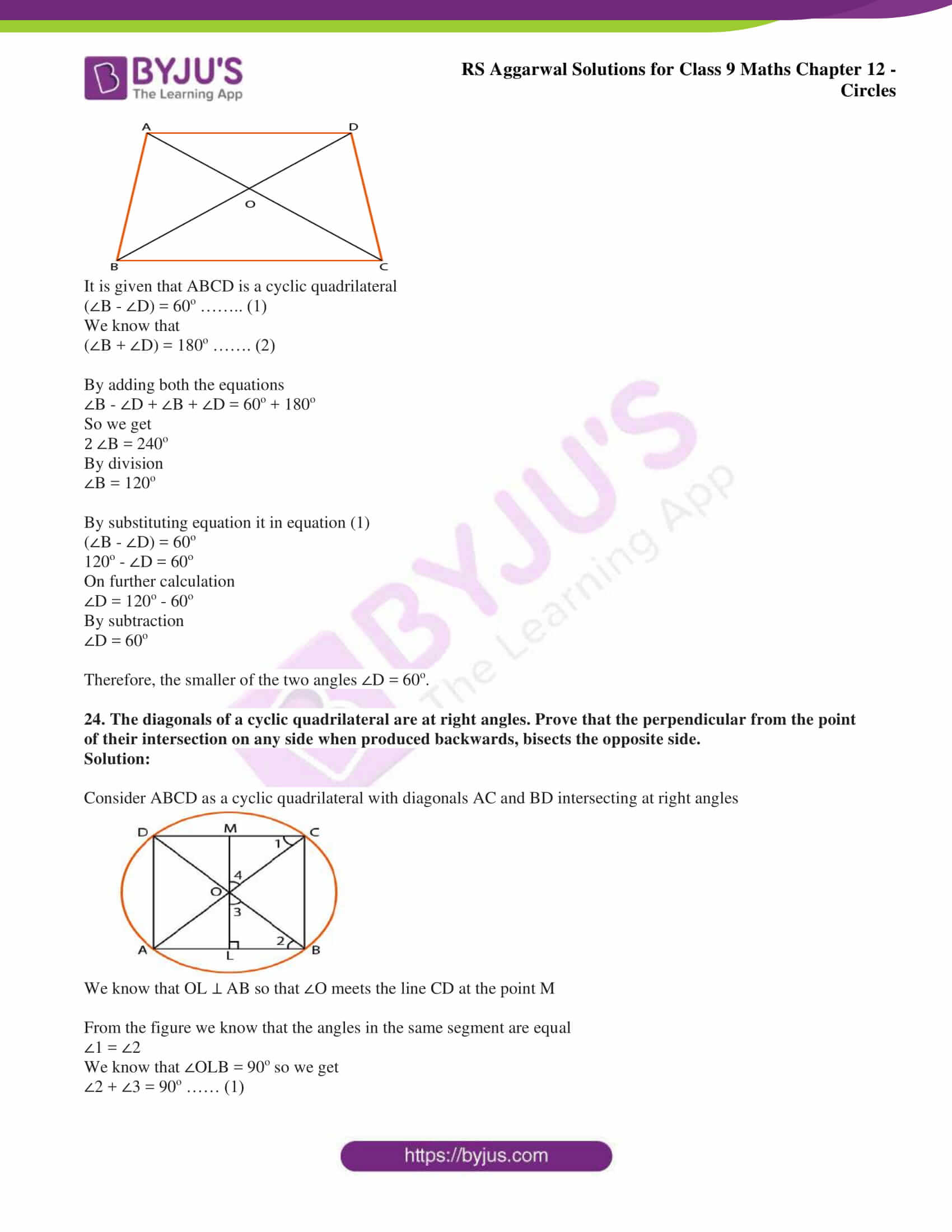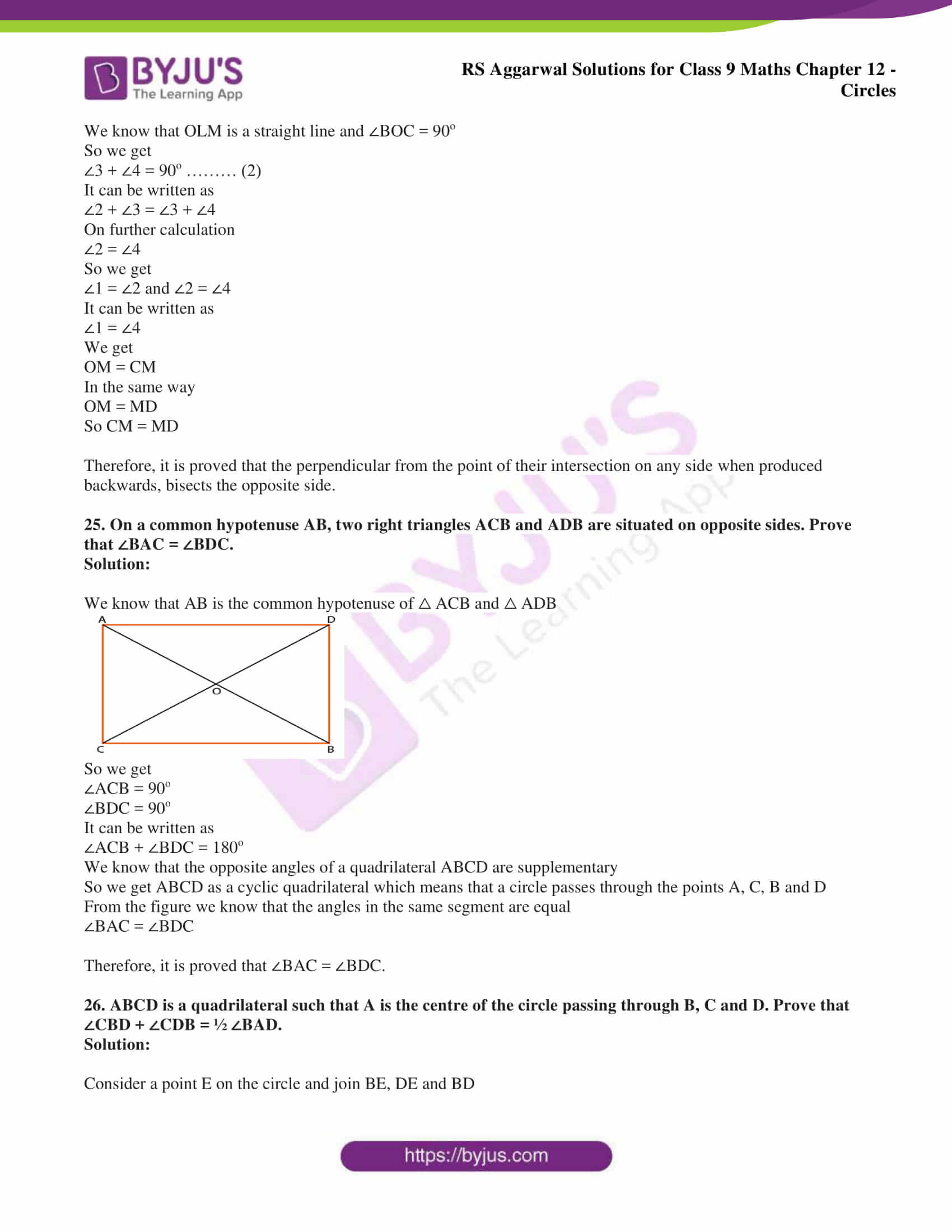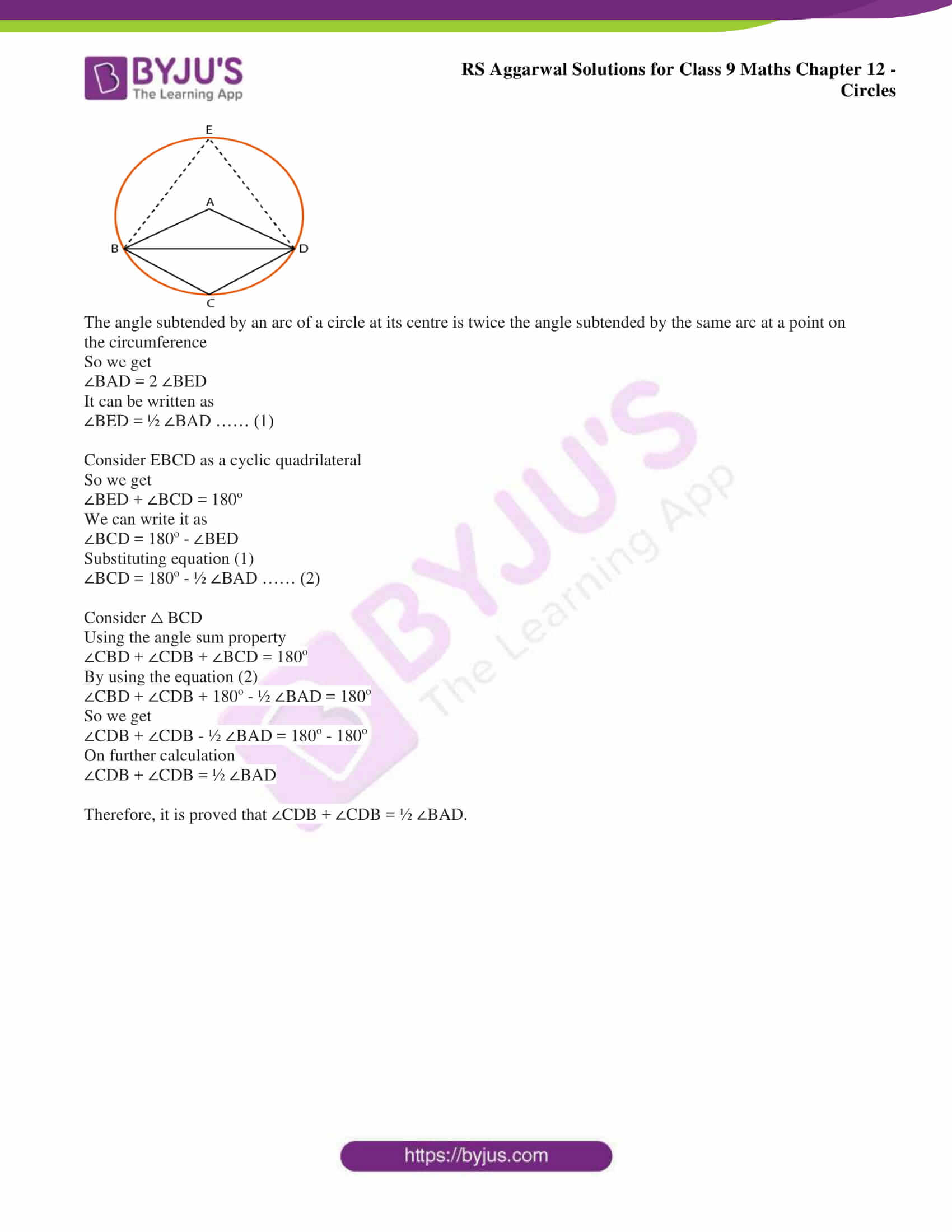## Access RS Aggarwal Solutions for Class 9 Chapter 12: Circles Exercise 12C

### Exercise 12(C) PAGE: 482

1. In the given figure, ABCD is a cyclic quadrilateral whose diagonals intersect at P such that ∠DBC = 60o and ∠BAC = 40o. Find

(i) ∠BCD,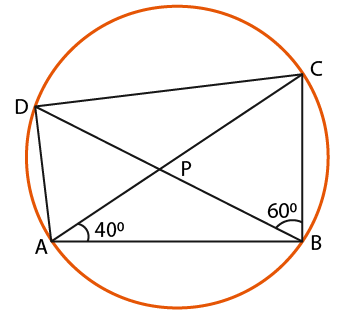Solution:

(i) We know that the angles in the same segment are equal

So we get

∠BDC = ∠BAC = 40o

Consider △ BCD

Using the angle sum property

∠BCD + ∠BDC + ∠DBC = 180o

By substituting the values

∠BCD + 40o + 60o = 180o

On further calculation

∠BCD = 180o – 40o – 60o

By subtraction

∠BCD = 180o – 100o

So we get

∠BCD = 80o

(ii) We know that the angles in the same segment are equal

So we get

2. In the given figure, POQ is a diameter and PQRS is a cyclic quadrilateral. If ∠PSR = 150o, find ∠RPQ.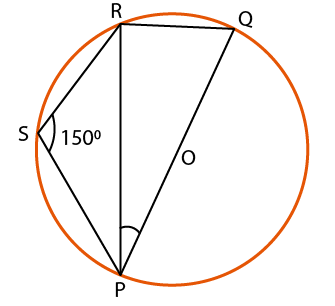Solution:

We know that PQRS is a cyclic quadrilateral

It can be written as

∠PSR+ ∠PQR = 180o

By substituting the values

150o + ∠PQR = 180o

On further calculation

∠PQR = 180o – 150o

By subtraction

∠PQR = 30o

We know that the angle in semi-circle is a right angle

∠PRQ = 90o

Consider △ PRQ

Using the angle sum property

∠PQR + ∠PRQ + ∠RPQ = 180o

By substituting the values

30o + 90o + ∠RPQ = 180o

On further calculation

∠RPQ = 180o – 30o – 90o

By subtraction

∠RPQ = 180o – 120o

So we get

∠RPQ = 60o

Therefore, ∠RPQ = 60o.

3. In the given figure, O is the centre of the circle and arc ABC subtends an angle of 130o at the centre. If AB is extended to P, find ∠PBC.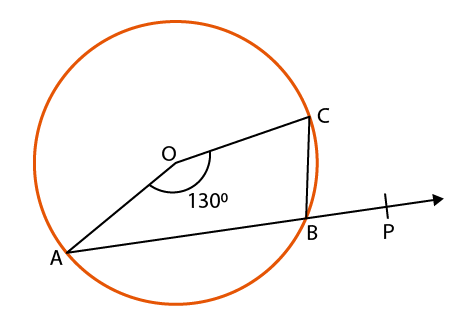Solution:

Consider a point D on the arc CA and join DC and AD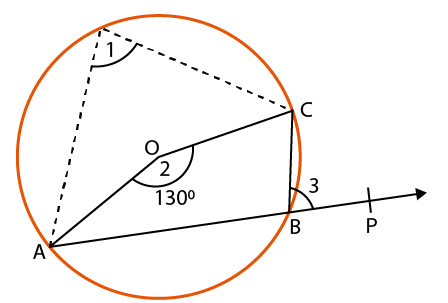We know that the angle subtended by an arc is twice the angle subtended by it on the circumference in the alternate segment

So we get

∠2 = 2 ∠1

By substituting the values

130o = 2 ∠1

So we get

∠1 = 65o

From the figure we know that the exterior angle of a cyclic quadrilateral = interior opposite angle

∠PBC = ∠1

So we get ∠PBC = 65o

Therefore, ∠PBC = 65o.

4. In the given figure, ABCD is a cyclic quadrilateral in which AE is drawn parallel to CD, and BA is produced to F. If ∠ABC = 92o and ∠FAE = 20o, find ∠BCD.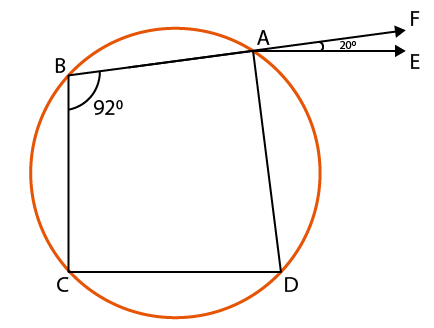Solution:

We know that ABCD is a cyclic quadrilateral

So we get

By substituting the values

On further calculation

By subtraction

We know that AE || CD

From the figure we know that

We know that the exterior angle of a cyclic quadrilateral = interior opposite angle

So we get

∠BCD = ∠DAF

We know that

It is given that ∠FAE = 20o

By substituting the values

∠BCD = 88o + 20o

∠BCD = 108o

Therefore, ∠BCD = 108o.

5. In the given figure, BD = DC and ∠CBD = 30o, find ∠BAC.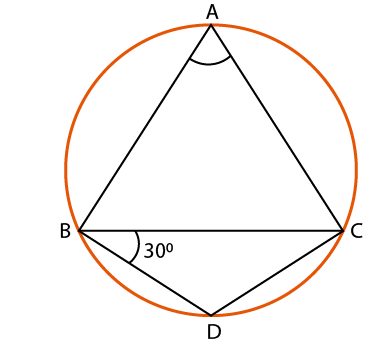Solution:

It is given that BD = DC

From the figure we know that

∠BCD = ∠CBD = 30o

Consider △ BCD

Using the angle sum property

∠BCD + ∠CBD + ∠CDB = 180o

By substituting the values

30o + 30o + ∠CDB = 180o

On further calculation

∠CDB = 180o – 30o – 30o

By subtraction

∠CDB = 180o – 60o

So we get

∠CDB = 120o

We know that the opposite angles of a cyclic quadrilateral are supplementary

It can be written as

∠CDB + ∠BAC = 180o

By substituting the values

120o + ∠BAC = 180o

On further calculation

∠BAC = 180o – 120o

By subtraction

∠BAC = 60o

Therefore, ∠BAC = 60o.

6. In the given figure, O is the centre of the given circle and measure of arc ABC is 100o. Determine ∠ADC and ∠ABC.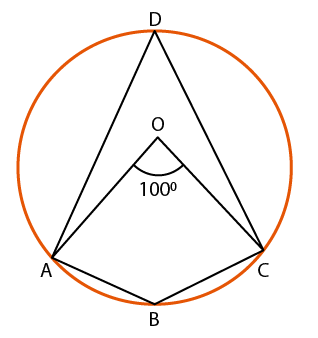Solution:

We know that the angle subtended by an arc is twice the angle subtended by it on the circumference

From the figure we know that

∠AOC = 100o

So we get

It can be written as

By substituting the values

So we get

We know that the opposite angles of a cyclic quadrilateral are supplementary

It can be written as

By substituting the values

50o + ∠ABC = 180o

On further calculation

∠ABC = 180o – 50o

By subtraction

∠ABC = 130o

Therefore, ∠ADC = 50o and ∠ABC = 130o.

7. In the given figure, △ ABC is equilateral. Find

(i) ∠BDC,

(ii) ∠BEC.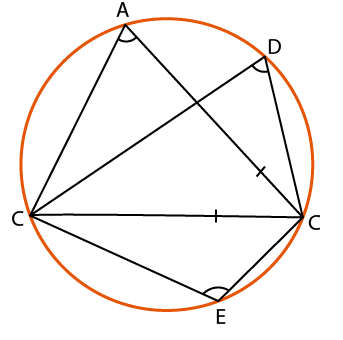Solution:

It is given that △ ABC is equilateral

We know that

∠BAC = ∠ABC = ∠ACB = 60o

(i) We know that the angles in the same segment of a circle are equal

∠BDC = ∠BAC = 60o

So we get

∠BDC = 60o

(ii) We know that the opposite angles of a cyclic quadrilateral are supplementary

So we get

∠BAC + ∠BEC = 180o

By substituting the values

60o + ∠BEC = 180o

On further calculation

∠BEC = 180o – 60o

By subtraction

∠BEC = 120o

8. In the adjoining figure, ABCD is a cyclic quadrilateral in which ∠BCD = 100o and ∠ABD = 50o. Find ∠ADB.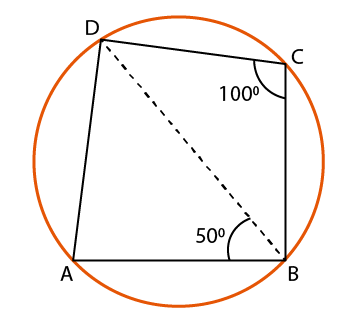Solution:

It is given that ABCD is a cyclic quadrilateral

We know that the opposite angles of a cyclic quadrilateral are supplementary

So we get

∠A + ∠C = 180o

By substituting the values

∠A + 100o = 180o

On further calculation

∠A = 180o – 100o

By subtraction

∠A = 80o

Consider △ ABD

Using the angle sum property

∠A + ∠ABD + ∠ADB = 180o

By substituting the values

80o + 50o + ∠ADB = 180o

On further calculation

∠ADB = 180o – 80o – 50o

By subtraction

So we get

9. In the given figure, O is the centre of a circle and ∠BOD =150o. Find the values of x and y.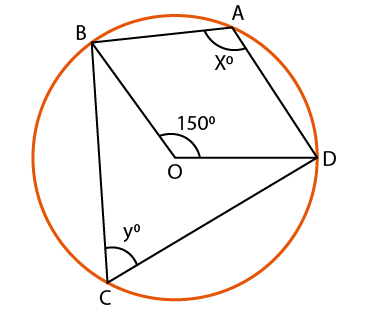Solution:

It is given that O is the centre of a circle and ∠BOD =150o

We know that

Reflex ∠BOD = (360o – ∠BOD)

By substituting the values

Reflex ∠BOD = (360o – 150o)

By subtraction

Reflex ∠BOD = 210o

Consider x = ½ (reflex ∠BOD)

By substituting the value

x = 210/2

So we get

x = 105o

We know that

x + y = 180o

By substituting the values

105o + y = 180o

On further calculation

y = 180o – 105o

By subtraction

y = 75o

Therefore, the value of x is 105o and y is 75o.

10. In the given figure, O is the centre of the circle and ∠DAB = 50o. Calculate the values of x and y.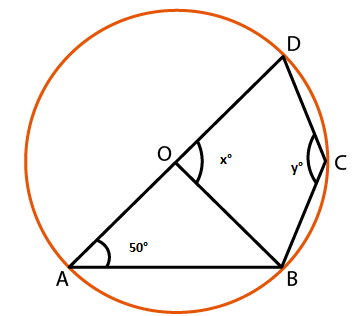Solution:

It is given that O is the centre of the circle and ∠DAB = 50o

We know that the radii of the circle are equal

OA = OB

From the figure we know that

∠OBA = ∠OAB = 50o

Consider △ OAB

Using the angle sum property

∠OAB + ∠OBA + ∠AOB = 180o

By substituting the values

50o + 50o + ∠AOB = 180o

On further calculation

∠AOB = 180o – 50o – 50o

By subtraction

∠AOB = 180o – 100o

So we get

∠AOB = 80o

From the figure we know that AOD is a straight line

It can be written as

x = 180o – ∠AOB

By substituting the values

x = 180o – 80o

By subtraction

x = 100o

We know that the opposite angles of a cyclic quadrilateral are supplementary

So we get

∠DAB + ∠BCD = 180o

By substituting the values

50o + ∠BCD = 180o

On further calculation

∠BCD = 180o – 50o

By subtraction

y = ∠BCD = 130o

Therefore, the value of x is 100o and y is 130o.

11. In the given figure, sides AD and AB of cyclic quadrilateral ABCD are produced to E and F respectively. If ∠CBF = 130o and ∠CDE = xo, find the value of x.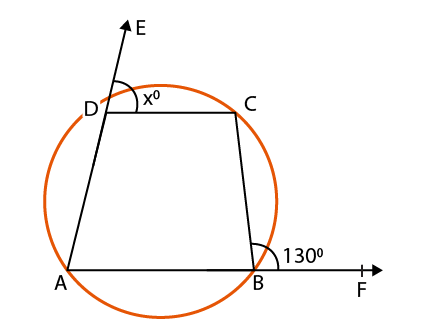Solution:

In a cyclic quadrilateral we know that the exterior angle is equal to the interior opposite angle

So we get

∠CBF = ∠CDA

It can be written as

130o = 180o – x

On further calculation

x = 180o – 130o

By subtraction

x = 50o

12. In the given figure, AB is a diameter of a circle with centre O and DO || CB. If ∠BCD = 120o, calculate

(ii) ∠ABD,

(iii) ∠CBD,

Also, show that △ AOD is an equilateral triangle.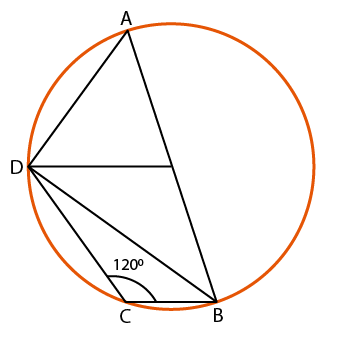Solution:

It is given that AB is a diameter of a circle with centre O and DO || CB

(i) We know that ABCD is a cyclic quadrilateral

It can be written as

By substituting the values

On further calculation

By subtraction

(ii) We know that the angle in a semi-circle is right angle

∠BDA = 90o

Consider △ ABD

By using the angle sum property

∠BDA + ∠BAD + ∠ABD = 180o

By substituting the values

90o + 60o + ∠ABD = 180o

On further calculation

∠ABD = 180o – 90o – 60o

By subtraction

∠ABD = 180o – 150o

So we get

∠ABD = 30o

(iii) We know that OD = OA

From the figure we know that

∠ODB + ∠ODA = 90o

By substituting the values

∠ODB + 60o = 90o

On further calculation

∠ODB = 90o – 60o

By subtraction

∠ODB = 30o

It is given that DO || CB

We know that the alternate angles are equal

∠CDB = ∠ODB = 30o

(iv) From the figure we know that

By substituting the values

Consider △ AOD

By using the angle sum property

∠ODA + ∠OAD + ∠AOD = 180o

By substituting the values

60o + 60o + ∠AOD = 180o

On further calculation

∠AOD = 180o – 60o – 60o

By subtraction

∠AOD = 180o – 120o

So we get

∠AOD = 60o

We know that all the angles of the △ AOD is 60o

Therefore, it is proved that △ AOD is an equilateral triangle.

13. Two chords AB and CD of a circle intersect each other at P outside the circle. If AB = 6cm, BP = 2cm and PD = 2.5cm, find CD.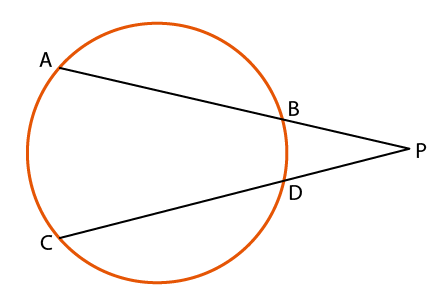Solution:

It is given that AB and CD of a circle intersect each other at P outside the circle. If AB = 6cm, BP = 2cm and PD = 2.5cm

So we get

AP × BP = CP × DP

From the figure we know that CP = CD + DP

By substituting the values

8 × 2 = (CD + 2.5) × 2.5 cm

Consider x = CD

So we get

8 × 2 = (x + 2.5) × 2.5

On further calculation

16 = 2.5x + 6.25

It can be written as

2.5x = 16 – 6.25

By subtraction

2.5x = 9.75

By division

x = 9.75/2.5

So we get

x = 3.9cm

Therefore, CD = 3.9cm.

14. In the given figure, O is the centre of a circle. If ∠AOD = 140o and ∠CAB = 50o, calculate

(i) ∠EDB,

(ii) ∠EBD.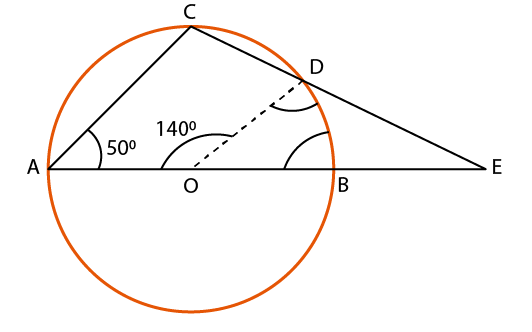Solution:

(i) We know that

∠BOD + ∠AOD = 180o

By substituting the values

∠BOD + 140o = 180o

On further calculation

∠BOD = 180o – 140o

By subtraction

∠BOD = 40o

We know that OB = OD

So we get ∠OBD = ∠ODB

Consider △ OBD

By using the angle sum property

∠BOD + ∠OBD + ∠ODB = 180o

We know that ∠OBD = ∠ODB

So we get

40o + 2 ∠OBD = 180o

On further calculation

2 ∠OBD = 180o – 40o

By subtraction

2 ∠OBD = 140o

By division

∠OBD = 70o

We know that ABCD is a cyclic quadrilateral

∠CAB + ∠BDC = 180o

∠CAB + ∠ODB + ∠ODC = 180o

By substituting the values

50o + 70o + ∠ODC = 180o

On further calculation

∠ODC = 180o – 50o – 70o

By subtraction

∠ODC = 180o – 120o

So we get

∠ODC = 60o

Using the angle sum property

∠EDB + ∠ODC + ∠ODB = 180o

By substituting the values

∠EDB + 60o + 70o = 180o

On further calculation

∠EDB = 180o – 60o – 70o

By subtraction

∠EDB = 180o – 130o

So we get

∠EDB = 50o

(ii) We know that

∠EDB + ∠OBD = 180o

By substituting the values

∠EDB + 70o = 180o

On further calculation

∠EDB = 180o – 70o

By subtraction

∠EDB = 110o

15. In the given figure, △ ABC is an isosceles triangle in which AB = AC and a circle passing through B and C intersects AB and AC at D and E respectively. Prove that DE || BC.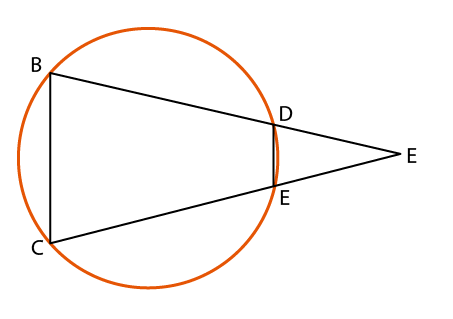Solution:

We know that △ ABC is an isosceles triangle in which AB = AC and a circle passing through B and C intersects AB and AC at D and E

So AB = AC

We get

∠ACB = ∠ABC

It can be written as

So we get

DE || BC

Therefore, it is proved that DE || BC.

16. In the given figure, AB and CD are two parallel chords of a circle. If BDE and ACE are straight lines, intersecting at E, prove that △ AEB is isosceles.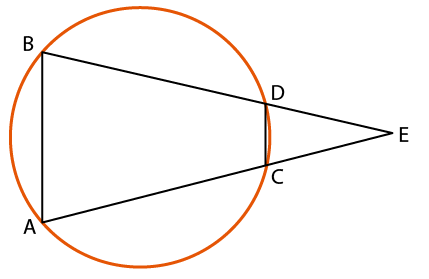Solution:

It is given that AB and CD are two parallel chords of a circle. If BDE and ACE are straight lines, intersecting at E

We know that in a cyclic quadrilateral exterior angle is equal to the interior opposite angle.

It can be written as

Exterior ∠EDC = ∠A

Exterior ∠DCE = ∠B

We know that AB || CD

So we get

∠EDC = ∠B and ∠DCE = ∠A

We get

∠A = ∠B

Therefore, it is proved that △ AEB is isosceles.

17. In the given figure, ∠BAD = 75o, ∠DCF = xo and ∠DEF = yo. Find the values of x and y.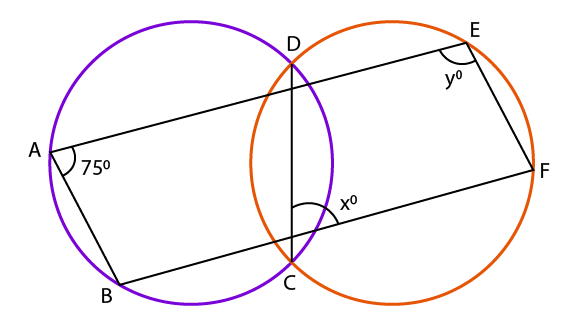Solution:

In a cyclic quadrilateral we know that the exterior angle is equal to the interior opposite angle

So we get

It can be written as

∠DCF = x = 75o

We get x = 75o

We know that the opposite angles of a cyclic quadrilateral is 180o

So we get

∠DCF + ∠DEF = 180o

By substituting the values

75o + ∠DEF = 180o

On further calculation

∠DEF = 180o – 75o

By subtraction

∠DEF = y = 105o

Therefore, the values of x and y is 75o and 105o.

18. In the given figure, ABCD is a quadrilateral in which AD = BC and ∠ADC = ∠BCD. Show that the points A, B, C, D lie on a circle.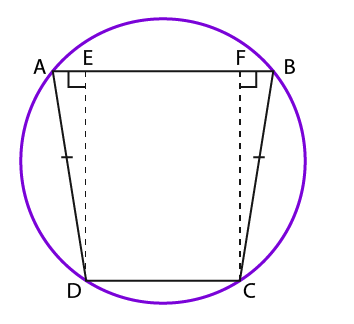Solution:

It is given that ABCD is a quadrilateral in which AD = BC and ∠ADC = ∠BCD

Construct DE ⊥ AB and CF ⊥ AB

Consider △ ADE and △ BCF

We know that

∠AED + ∠BFC = 90o

From the figure it can be written as

It is given that

By AAS congruence criterion

∠A = ∠B (c. p. c. t)

We know that the sum of all the angles of a quadrilateral is 360o

∠A + ∠B + ∠C + ∠D = 360o

By substituting the values

2 ∠B + 2 ∠D = 360o

By taking 2 as common

2 (∠B + ∠D) = 360o

By division

∠B + ∠D = 360/2

So we get

∠B + ∠D = 180o

So, ABCD is a cyclic quadrilateral.

Therefore, it is proved that the points A, B, C and D lie on a circle.

19. Prove that the perpendicular bisectors of the sides of a cyclic quadrilateral are concurrent.

Solution: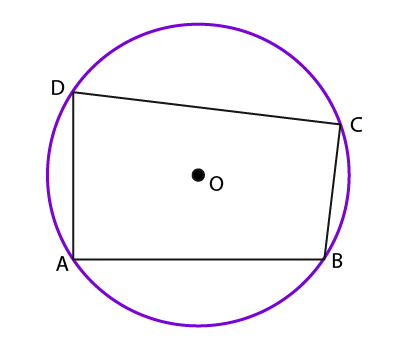Consider ABCD as a cyclic quadrilateral with centre O passing through the points A, B, C, D

We know that AB, BC, CD and DA are the chords of the circle and its right bisector passing through the centre O

So we know that the right bisectors of AB, BC, CD and DA pass through the centre O and are concurrent.

Therefore, it is proved that the perpendicular bisectors of the sides of a cyclic quadrilateral are concurrent.

20. Prove that the circles described with the four sides of a rhombus as diameters pass through the point of intersection of its diagonals.

Solution: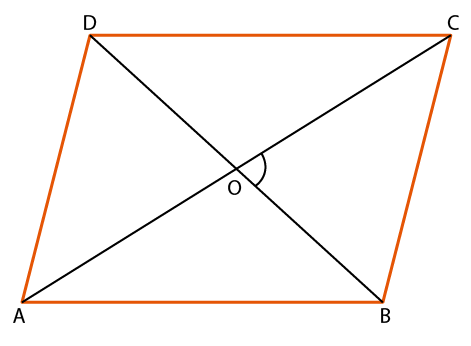Consider ABCD as a rhombus

We know that the diagonals AC and BD intersect at the point O

From the figure we know that the diagonals of the rhombus bisect at right angles

It can be written as

∠BOC = 90o

Thus, ∠BOC lies in the circle

We know that the circle can be drawn with BC as the diameter having the centre O

In the same way, all the circles with AB, AD and CD as diameters will pass through the centre O.

Therefore, it is proved that the circles described with the four sides of a rhombus as diameters pass through the point of intersection of its diagonals.

21. ABCD is a rectangle. Prove that the centre of the circle through A, B, C, D is the point of intersection of its diagonals.

Solution: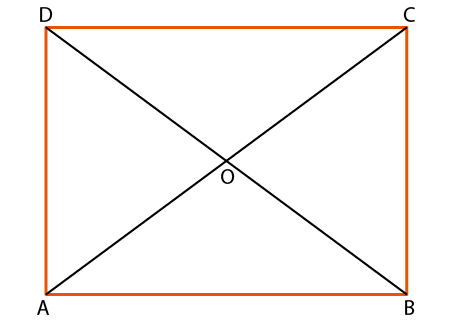Consider ABCD as a rectangle

We know that O is the point of intersection of the diagonals AC and BD

The diagonals of a rectangle are equal and bisect each other

So we get

OA = OB = OC = OD

We get O as the centre of the circle through A, B, C and D

Therefore, it is proved that the centre of the circle through A, B, C, D is the point of intersection of its diagonals.

22. Give a geometrical construction for finding the fourth point lying on a circle passing through three given points, without finding the centre of the circle. Justify the construction.

Solution:

Consider A, B, C as the points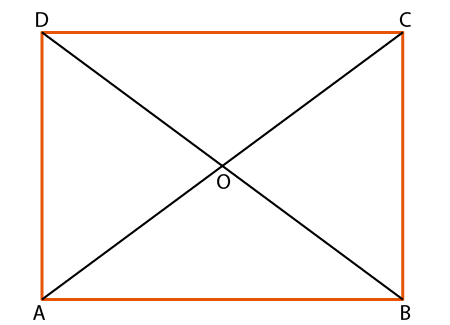Taking B as the centre and radius equal to AC construct an arc

Taking C as the centre and radius equal to AB construct another arc which cuts the arc at D

So we get D as the required point BD and CD

Consider △ ABC and △ DCB

We know that

AB = DC and AC = DB

BC and CB are common i.e. BC = CB

By SSS congruence criterion

△ ABC ≅ △ DCB

∠BAC = ∠CDB (c. p. c. t)

We know that BC subtends equal angles ∠BAC and ∠CDB on the same side

So we get A, B, C, D are cyclic.

Therefore, the points A, B, C, D are cyclic.

23. In a cyclic quadrilateral ABCD, if (∠B – ∠D) = 60o, show that the smaller of the two is 60o.

Solution: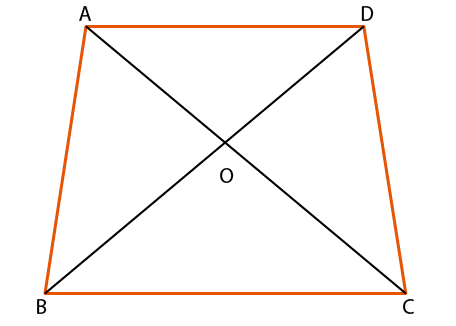It is given that ABCD is a cyclic quadrilateral

(∠B – ∠D) = 60o …….. (1)

We know that

(∠B + ∠D) = 180o ……. (2)

∠B – ∠D + ∠B + ∠D = 60o + 180o

So we get

2 ∠B = 240o

By division

∠B = 120o

By substituting equation it in equation (1)

(∠B – ∠D) = 60o

120o – ∠D = 60o

On further calculation

∠D = 120o – 60o

By subtraction

∠D = 60o

Therefore, the smaller of the two angles ∠D = 60o.

24. The diagonals of a cyclic quadrilateral are at right angles. Prove that the perpendicular from the point of their intersection on any side when produced backwards, bisects the opposite side.

Solution:

Consider ABCD as a cyclic quadrilateral with diagonals AC and BD intersecting at right angles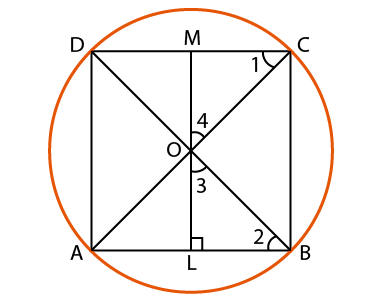We know that OL ⊥ AB so that ∠O meets the line CD at the point M

From the figure we know that the angles in the same segment are equal

∠1 = ∠2

We know that ∠OLB = 90o so we get

∠2 + ∠3 = 90o …… (1)

We know that OLM is a straight line and ∠BOC = 90o

So we get

∠3 + ∠4 = 90o ……… (2)

It can be written as

∠2 + ∠3 = ∠3 + ∠4

On further calculation

∠2 = ∠4

So we get

∠1 = ∠2 and ∠2 = ∠4

It can be written as

∠1 = ∠4

We get

OM = CM

In the same way

OM = MD

So CM = MD

Therefore, it is proved that the perpendicular from the point of their intersection on any side when produced backwards, bisects the opposite side.

25. On a common hypotenuse AB, two right triangles ACB and ADB are situated on opposite sides. Prove that ∠BAC = ∠BDC.

Solution:

We know that AB is the common hypotenuse of △ ACB and △ ADB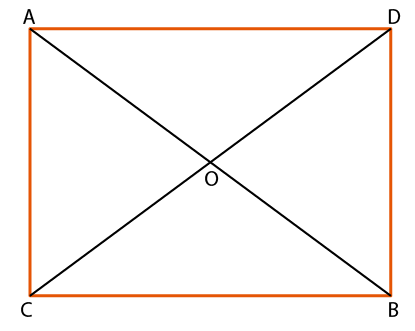So we get

∠ACB = 90o

∠BDC = 90o

It can be written as

∠ACB + ∠BDC = 180o

We know that the opposite angles of a quadrilateral ABCD are supplementary

So we get ABCD as a cyclic quadrilateral which means that a circle passes through the points A, C, B and D

From the figure we know that the angles in the same segment are equal

∠BAC = ∠BDC

Therefore, it is proved that ∠BAC = ∠BDC.

26. ABCD is a quadrilateral such that A is the centre of the circle passing through B, C and D. Prove that ∠CBD + ∠CDB = ½ ∠BAD.

Solution:

Consider a point E on the circle and join BE, DE and BD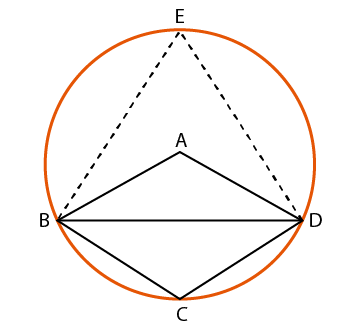The angle subtended by an arc of a circle at its centre is twice the angle subtended by the same arc at a point on the circumference

So we get

It can be written as

∠BED = ½ ∠BAD …… (1)

Consider EBCD as a cyclic quadrilateral

So we get

∠BED + ∠BCD = 180o

We can write it as

∠BCD = 180o – ∠BED

Substituting equation (1)

∠BCD = 180o – ½ ∠BAD …… (2)

Consider △ BCD

Using the angle sum property

∠CBD + ∠CDB + ∠BCD = 180o

By using the equation (2)

∠CBD + ∠CDB + 180o – ½ ∠BAD = 180o

So we get

∠CDB + ∠CDB – ½ ∠BAD = 180o – 180o

On further calculation

∠CDB + ∠CDB = ½ ∠BAD

Therefore, it is proved that ∠CDB + ∠CDB = ½ ∠BAD.

### Access other exercise solutions of Class 9 Maths Chapter 12: Circles

Exercise 12A Solutions 23 Questions

Exercise 12B Solutions 18 Questions

### RS Aggarwal Solutions Class 9 Maths Chapter 12 – Circles Exercise 12C

RS Aggarwal Solutions Class 9 Maths Chapter 12 Circles Exercise 12C is the third exercise which contains problems solved using theorems and some of the results on cyclic quadrilaterals.

### Key features of RS Aggarwal Solutions for Class 9 Maths Chapter 12: Circles Exercise 12C

• The RS Aggarwal solutions are primarily based on frequently asked questions in the board exam.
• The solutions are explained in simple language based on CBSE guidelines and exam pattern.
• The PDF of solutions can be used by the students as a vital resource to boost their exam preparation.
• Comprehensive explanation of solutions mainly help to improve conceptual knowledge among students.